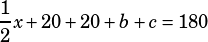Can you please explain College Board Test 5 Section 4 #36?

I understand that angle A is half of x- the only part of the college board explanation I’m not understanding is why we do (360-x) while setting up the equation. Thanks!

Sure. Without looking at CB’s solution, here’s how I do it.First, as you pointed out, the measure of angle A is half of . That’ll be useful. The other thing I think is useful is to draw segment BC and mark the angles you create (I’ll say their measures are  and ). Now you’ve got two triangles, ABC and PBC.Each triangle, of course, has angles that add up to 180°. You can write two equations that show this:

Triangle ABC:Triangle PBC:All you need to do to solve for x is set those left sides equal to each other since they both equal 180 (b and c will cancel right out!).